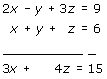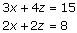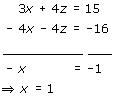## Definition Of Elimination Method

Elimination Method is the process of eliminating one of the variables in a system of equations using addition or subtraction in conjunction with multiplication or division and solving the system of equations.

### Solved Example on Elimination Method

#### Ques: Using the elimination method, solve the system of equations. 2x - y + 3z = 9 x + y + z = 6 x - y + z = 2

##### Choices:

A. (2, 1, -1)
B. (4, 1, 1)
C. (1, -1, 2)
D. (1, 2, 3)
Step 1:[Add the first two equations to eliminate y.]
Step 2:[Add the second and third equations to eliminate y.]
Step 3: We now have a system of two equations in two variables:Step 4:[Multiply the second equation with - 2 and add, and then solve for x.]